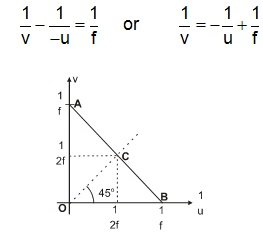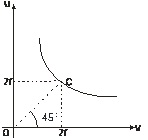# Find the focal length of the convex lens using uv  method

3 years ago

The relation between u, v and f for a convex lens is,

v-1 + u-1 = f-1

Using the proper sign convention, u is negative, u negative, v and f are positive. So we have,Comparing with y = mx + c, graph between v-1 & u-1  is negative with slope –1 and intercept  f-1 .The corresponding graph is as shown in figure.

Proceeding in the similar manner as discussed in case of a concave mirror the focal length of the lens can be calculated by measuring the coordinates of either of the points A, B and C.u–v Graph
The u versus v graph is a hyperbola as shown in figure. By measuring the coordinates of point C whose coordinates are (2f, 2f) we can calculate the focal length of the lens.